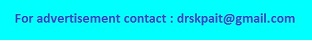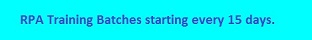### Acoustic Impedance Calculation

The calculator will calculate the Acoustic Impedance of material for given values of density and velocity. The acoustic impedance can be defined as the product of its density and acoustic velocity. It is important in determining the transmission and reflection at the boundary of two materials having different impedances.
Formula: Z = p * V
Where, Z - Acoustic Impedance of material, p - density, V - Velocity
 Density(kg/m3): Velocity(m/s): Acoustic Impedance: (Ns/m3)
Ultrasonic pulse or sound can travel through a materials depends on a property of the material called acoustic impedance which is denoted by Z.The travelling through material with the influence of the sound pressure because atoms of solid are are elastically bound and the excess pressure results in a wave propagating through the solid. The significance of acoustic impedance in assessing absorption of sound in a medium and in the design of ultrasonic transducers.